# Thesis – Chapter 2: Experimental Setup and Overview of the Temperature Filament

### Experimental Setup and Overview of the Temperature Filament

#### Large Plasma Device

The Large Plasma Device (LAPD-U), part of the Basic Plasma Science Facility (BaPSF) at UCLA, provides an ideal parameter regime in which to perform this experimental investigation. The present device, as shown in Fig. 2.1, is a larger version of the one described in Gekelman et al. .

The LAPD-U produces plasma by discharging a cathode-anode pair. A barium-oxide coated, nickel cathode is heated to emission temperature near 850 °C. A wire mesh molybdenum anode is positioned 50 cm away from the cathode. A large capacitor bank connects the pair and discharges a few thousand Amperes of current for some fixed bias voltage (typically in the 65 V range). The anode mesh is 50% transparent, allowing half of the electrons emitted by the cathode to pass through and travel down the length of the device. This produces plasmas that fill the 20 meter-long vacuum chamber. During the main discharge (a flat-top current pulse between the cathode-anode pair lasting up to 12 ms), the resulting plasma column extends up to 60 centimeters in diameter.

The LAPD-U provides a wide range of plasma parameters for study. The solenoidal magnetic field may be set to any value between 500 and 2500 Gauss. Magnetic coil elements are separately connected, allowing for various field configurations (mirror, beach, etc.). Inert gases are typically used to generate plasmas since these do not chemically interact with the oxide coating of the cathode. Table 2.1 lists typical plasma parameters for the LAPD-U.

#### Temperature Filament Setup

##### Afterglow PlasmaFigure 2.2: Time evolution of the discharge current through the anode-cathode pair (black,$I_{dis}$) and the line-averaged column electron density (red,$n_e$) during an LAPD-U plasma-pulse.

The principal results of this thesis are obtained in the afterglow phase of the LAPD-U plasma. The afterglow is a common term used to describe the plasma that remains in the vacuum chamber after a cathode discharge has been turned off. Figure 2.2 is a plot of the LAPD-U discharge current (black trace) and the line-averaged electron density (red trace) as measured by an interferometer. A double-sided blue arrow extends across the post-discharge regime in which the plasma is in the afterglow phase. When the discharge shuts off there is no longer a heating source to maintain plasma temperature. Thermal conduction to the ends of the device causes the electron temperature to decay rapidly, on the order of microseconds. Diffusion is a much slower processes, however, so the plasma density decays on the order of milliseconds. As a result, the LAPD-U afterglow plasma features a very low temperature ($T_e, T_i < 1$ eV) while maintaining a moderate density ($n_e \approx 1 \times 10^{12}$
cm-3) for the first 30 ms after the primary discharge ends. Most of the temperature filament experiments begin at a time 0.5 ms after the discharge current ends and last for 10 to 15 ms.

##### Basic Overview

A small heat source is applied at one end of the LAPD-U during the early afterglow phase in order to create a temperature filament that is approximately five times hotter than the surrounding background. Plasma properties are measured throughout the resulting three dimensional structure over time scales that allow the relevant transport and turbulence behaviors to arise.

A schematic of this system (not to scale) is given in Fig. 2.3. A cylindrical coordinate system is shown and is referenced throughout this treatment. The axial coordinate is labeled z, the radial coordinate r, and the azimuthal coordinate is θ. The electron beam is located at$(r,z) = (0,0)$ cm. The heat source is modeled as a one meter long, 3 mm across rigid cylinder of hot plasma that is placed 16 m away from the main cathode/anode. The heating done in this region is classically transported to setup the filament structure that may reach 12 m in length and 1 cm in diameter. Further details of this system are presented in the following sections.

##### Heating Source: Lanthanum Hexaboride Electron Beam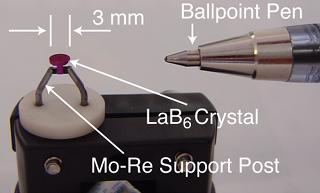Figure 2.4: Photograph of the LaB6 electron beam. The beam is a single crystal of LaB6 permanently fixed in a mounting structure. The crystal is held in place by Mo-Re posts. A common ballpoint pen is provided for scale.

Plasma heating is accomplished by the injection of energetic electrons from a crystal of lanthanum hexaboride (LaB66 is low enough that direct heating of the crystal, accomplished by running current through it, provides adequate energy for thermionic emission. An operating temperature of 1800 °K is used. Such crystal temperatures are achieved by running a current through the crystal itself. Figure 2.4 shows the electron beam device as purchased from Applied Physics Technologies (a-p-tech.com): a single crystal of LaB6 is held in place above a ceramic washer by molybdenum-rhenium (Mo-Re) posts. On the underside of the washer, the posts provide the access point for driving the heater current.

The LaB6 crystal is mounted on the end of a standard LAPD-U probe shaft and placed in the plasma away from the main cathode/anode (drawn in Fig. 2.3). The main LAPD-U anode also serves as the anode for beam circuit, as shown in the diagram of Fig. 2.5. A heater current is continually run through the crystal to maintain emission temperature at all times during an experiment. The anode connection includes a pulse circuit that applies the beam voltage,$V_{beam}$, at a prescribed time within each LAPD-U discharge. The applied voltage set by$V_{beam}$ determines the energy of the emitted electrons, which allows the input heating power to be varied. Electron beam current is measured by monitoring the voltage across a 1 Ω resistor connected within the current path. Unless otherwise specified, the beam energy is 20 V for all experiments performed in helium. The ionization energy of helium is approximately 24.6 eV, which allows these experiments to be conducted with minimal concern for density production from the injected electrons. Some experiments are conducted at energies above 24.6 V, but these are not presented as part of the heat transport study.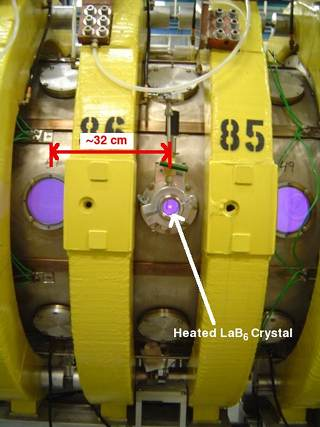Figure 2.6: The LaB6 crystal is seen glowing white hot (center) during a discharge pulse in the LAPD-U. The purple background seen inside the small windows is the typical LAPD-U helium plasma.

Figure 2.6 shows the heated crystal embedded in a plasma discharge. The distance between the yellow magnet coils is approximately 32 cm.

##### Diagnostics

The length of the LAPD-U plasma along the applied background magnetic field is approximately 16.6 m. Diagnostic ports are placed every 32 cm along this entire length, allowing for excellent axial resolution in this transport experiment.

Langmuir probes are used because they provide excellent spatial resolution (determined by the size of the probe) and time resolution (limited by the acquisition electronics). Furthermore, probes made out of nearly any metal can withstand the 5 eV plasma temperature throughout an entire experimental run while accessing the entire plasma volume of the device. The probes utilized in this study are made of either tantalum or nickel. Spatial access is provided by probe drives that move the probes in a full two-dimensional plane at any one axial position. Further details of Langmuir probe usage will be presented immediately preceding the display of results so that the method used to obtain the measurement may be explained in context.

In addition to the fundamental quantities of temperature, density, and flow velocity, the Langmuir probes are used to measure ion saturation current,$I_{sat}$. Ion saturation current is related to electron temperature and density by$I_{sat} \propto n_e\sqrt{T_e}$, meaning that fluctuations in these quantities are mixed by an$I_{sat}$ diagnostic. It is shown later that the coherent modes are accurately represented by an $laex I_{sat}$ measurement in situations where pure density or temperature measurements are not possible.

##### Measuring Electron Temperature

Swept Probe Technique

Electron temperature measurements using Langmuir probes most commonly employ the sweep method. Varying the applied voltage to a probe, V, while recording the current collected, I, results in a current versus voltage characteristic, the I-V curve, that can be fit to determine the electron temperature, electron density and plasma potential. This method is widely known and has been explained in the plasma physics literature (see Ch. 2 of Hutchinson ).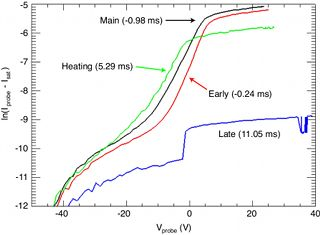Figure 2.7: Traces of the I-V characteristic are presented from the four distinct regions of plasma heating. The main discharge (black) represents the standard LAPD-U plasma as generated by the cathode-anode system. The early afterglow (red) occurs after the main discharge has ended, but before the beam heating begins. The heating stage (green) represents the primary experiment during which the temperature filament is maintained. Finally, the late afterglow (blue) represents the stage occurring after the beam heating has ended.

One problem in applying the swept probe method in fluctuation studies is that the parameters obtained represent averages over the time of the complete voltage sweep. A useful measurement of any wave requires the sweep to be performed at well over twice the frequency of the wave so that the Nyquist frequency of the resulting data also remains above that of the wave. A reproducible and established system for producing such a sweep (with frequency > 50 kHz) is difficult to build due to capacitive effects. An alternate method for measuring electron temperature using the I-V curve is developed.

From$I_{sat}$ measurements it is apparent that the plasma discharge and resulting temperature filament are highly reproducible. This technique involves maintaining a fixed probe voltage for a series of 20 discharges and then incrementing the voltage for the next 20 discharge series. After repeating this procedure for 100 voltages, an ensemble of 2000 discharges is used to construct an I-V characteristic corresponding to every time point of the acquisition. These characteristics allow for the calculation of plasma parameters with time resolution equivalent to that of the common$I_{sat}$ data sets.

Sample I-V traces are shown in Fig. 2.7. Traces from four different time periods are plotted because the voltage range over which the collected current is exponential (i.e., the range over which a fit is inversely proportional to the electron temperature) is found to vary with time. In order to automate the analysis of thousands of data signals it is necessary to determine the voltage range over which the temperature fits should be performed. This required manually examining selected traces from each probe position. The relevant voltage range is different for each region: main LAPD-U discharge (black), early afterglow without beam heating (red), beam heating (green), and late afterglow after the beam is shut off (blue). Electron temperature is calculated through linear fitting of the types of curves shown here, generally within the range$-15 \leq V_{probe} \leq 5$ V.

Calculation of the temperature is not possible in the late afterglow because the exponential range falls between two of the recorded voltages. There is no possibility of an accurate linear fit since the voltage range is smaller than the voltage resolution of the sweep.

An electron temperature measurement acquired from the swept probe technique is shown in Fig. 2.8 for the radial center of the filament. There is no direct filtering of this trace, though fluctuations are reduced by the ensemble nature of the data collection. The steady-state temperature reached in the later half of the time series is also observed with the companion technique for measuring electron temperature, the triple probe technique.

Triple Probe Technique

Electron temperature measurements are also made with triple probes in this thesis. The triple probe technique [Chen and Sekiguchi, 1965] uses three probe tips to simultaneously observe different regions of the I-V characteristic. This provides a continuous measure of temperature, floating potential and ion saturation current. Figure 2.9 shows the three tips of one of the triple probes used. These are 1 mm diameter discs embedded in a low-outgassing epoxy that is plasma facing.

Figure 2.10 is a circuit diagram illustrating the connections between the tips. Tips 1 and 2 are connected in fashion typical of$I_{sat}$ measurements. A fixed bias is applied between them and the voltage across a resistor in series is acquired. The unique aspect of the triple probe is that tip 3 is used to measure floating potential,$V_f$. The potential between the tip measuring$V_f$ and the electron collecting tip (2) is labeled as$V_{Te}$ and is related to the temperature according to,$T_e = V_{Te} / \ln(2)$ (Eq. 2.1), which is also derived from Chen and Sekiguchi, .Figure 2.10: Circuit diagram of the triple probe system. The probe tips are numbered and the measurement points are labeled according to whether they record electron temperature ($V_{Te}$), floating potential ($V_f$), or the voltage across the resistor corresponding to ion saturation current ($V_R$).

An example temperature trace from a triple probe measurement is presented in Fig. 2.11. This result is in qualitative agreement with the swept probe result of Fig. 2.8, even though the measurements are performed in different parameter regimes within different experimental run days. Both temperature traces identify the low frequency, coherent oscillations of the thermal wave (seen at t = 5 ms in Fig. 2.11) and a nearly steady-state temperature during the latter half of the heating cycle. Differences between these two results stem from the different background parameters (neutral fill pressure, axial position, heating power, etc.). The agreement between these very different methods suggests that both are valid in studying the filamentary system.

##### 2.2.6 Measuring Parallel Flow

The plasma flow measurements employ the Mach probe method of comparing$I_{sat}$ collection on probe faces oriented in opposite directions. That is, the surface normals of the two probe collection areas point in opposite directions. For two such probe faces, the parallel flow Mach number,$M_\parallel$, is (see page 85 of Hutchinson ),$M_\parallel = 0.45 \ln\left(\frac{I_{sat,1}}{I_{sat,2}}\right) \; (2.2)$

where 1 and 2 denote the probe faces and the Mach number is defined as$M = v/C_s$ where$C_s$ is the ion sound speed,$C_s = 9.79 \times 10^5 \left(\frac{\gamma ZT_e}{\mu}\right)^{-1/2} \;\text{(cm/s)} \; (2.3)$

in which γ is the adiabatic index, Z is the charge factor, and μ is the ion mass factor.Figure 2.11: Electron temperature as measured using the triple probe technique at the filament center and axial position z = 384 cm. While this is a different parameter regime compared to the swept probe measurement of Fig. 2.8, the result is qualitatively similar with respect to the detection of thermal waves and a steady-state temperature at the end of the heating cycle.

It should be noted that parallel flow results presented here are likely to be underestimates of the actual value. The factor of 0.45 given in Eq. 2.2 may be further modified due to the small size of the Langmuir probe tips compared to the ion gyroradius [Shikama et al., 2005]. Errors associated with probe measurements due to calculation of the effective collection area and pitch-angle with respect to the background magnetic field prevent a significant improvement in the accuracy of the reported flow Mach numbers. Regardless, the qualitative behavior of these results serves as a useful motivation for future efforts.

This analysis can be applied to probe faces with any orientation to the magnetic field, though the parallel orientation is the simplest theoretically. Perpendicular flows are difficult to measure in the temperature filament experiment through the Mach probe method because a probe with full radial and azimuthal diagnostic capability is also large enough to cause a disruptive perturbation. Parallel velocity measurements are made with Langmuir probes featuring the smallest possible surface area. In most cases this is a one millimeter diameter disk or a rectangular tip with the largest dimension on the order of one millimeter.

A limitation of this measurement is that it provides scaled information about the flow velocity with respect to the ion sound speed instead of the absolute velocity. In some instances a temperature measurement is available for the same set of discharges and the calculation of an absolute velocity is possible. In most cases presented in this thesis, however, the Mach probe measurement is performed without an auxiliary temperature measurement and the study focuses on the presence of supersonic flows and their relation to the other processes of the system.

Figure 2.12 demonstrates the calculation of plasma flows using$I_{sat}$ measurements from a double sided, or Janus, probe. These measurements are made at (r,z) = (0,64) cm, which places the probe within the heating region of beam. Recall that the heating source of this experiment is modeled as a one meter long, three millimeter wide cylindrical plasma made hot by the electrons emitted from the LaB6 crystal. The orientation of the probe tips in the figure is labeled according to which end of the LAPD-U they face. One tip, the$I_{sat}$ Beam Facing tip, faces the LaB6 crystal. The other tip, the$I_{sat}$ Anode Facing tip, faces the main anode of the LAPD-U. Flow analysis is performed with the anode facing signal in the denominator of Eq. 2.2, meaning that positive flow values correspond to plasma flowing toward the main LAPD-U anode. This convention is chosen because we expect the flow to dominate in this direction since the pressure gradient source is located at the opposite end of the device.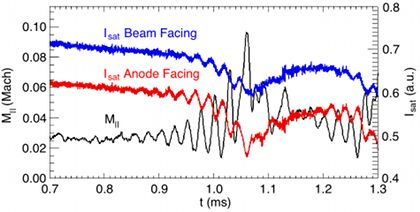Figure 2.12: (r,z) = (0,64) cm,$B_o = 900$ G. The$I_{sat}$ traces from both sides of a Janus probe (red and blue) are plotted in comparison to the parallel flow (black) calculated from them.

Coherent oscillations in the parallel flow are observed in Fig. 2.12. From the raw$I_{sat}$ traces it can be seen that the flow oscillations do not simply mirror the $I_{sat}$ measurements. In particular, the pulse-like event at t = 1.06 ms results from the large dip in$I_{sat}$ measured on the anode facing tip and the lack of a similar reduction at the beam facing tip. Later in time at t = 1.18 ms a coherent oscillation appears on the anode face and is delayed on the beam face. This behavior results in the calculation of another oscillating flow.

##### 2.2.7 Transport Modeling

The previous work using this experimental geometry to verify the existence of classical heat transport compared measured results with those of a code that modeled classical transport (see Section IV. of Burke et al., [2000b]). A similar effort is incorporated into this thesis.

A thesis in plasma theory by Shi  is a companion to this experimental treatment. The transport code is implemented by Shi is based on the equations of Braginskii . This set of equations includes the plasma continuity equation, the momentum equation, and the power balance equations. After applying the restraints of quasi-neutrality and cylindrical geometry along with the boundary conditions of the present experiment, these equations reduce to,where$V_z$ is the flow velocity parallel to the background magnetic field,$R_{in}$ is a collision operator for ion-neutral collisions,$Q_b$ is the heat input from the electron beam,$\tau_e$ is the electron collision period, and$\sigma_n$ is the ion-neutral collision cross-section. The model provides expected classical behavior for the density, electron temperature, and parallel flow evolution. This thesis is concerned with the experimental aspects of the temperature filament environment. The thesis written by Shi is concerned with the theoretical issues of the temperature filament, including heat transport and non-linear interactions between drift-Alfvén waves. Various figures within this thesis will compare measurements with Shi’s model results.

#### 2.3 Filament Behavior

##### 2.3.1 Temporal Behavior

The control circuit of the electron beam allows both the heating start time and its duration to be adjusted. The temporal evolution of the temperature filament exhibits three stages, as illustrated by Fig. 2.13. The solid black curve in Fig. 2.13 corresponds to the electron temperature measured, using a small triple probe, at an axial distance 384 cm from the beam injector at the radial center of the filament. For this figure the strength of the confinement magnetic field is 900 G.Figure 2.13: Electron temperature and beam current at (r,z) = (0, 384) cm. For the heating case (solid black), the electron beam is activated 0.5 μs after the LAPD-U discharge ends. The heated filament reaches temperatures comparable to that of the main plasma and is much hotter than the background afterglow plasma (dashed red). In this representation the beam current (dotted blue) is an ensemble trace while the heating is from a single shot.

The injected beam current is represented in Fig. 2.13 by the dotted blue curve, with t = 0 corresponding to the time when beam injection starts. The dashed red curve shows the decay of the electron temperature in the absence of beam injection, i.e., during the afterglow. It is seen that the heat source supplied by the beam causes a significant increase in temperature. For an interval of about 2 ms after the beam injection begins, a quiescent temperature evolution is observed. This behavior corresponds quantitatively to that predicted by the classical theory of heat transport based on Coulomb collisions [Braginskii, 1965; Spitzer and Härm, 1953b], as has been documented extensively in previous work [Burke et al., 2000b, 1998]. A second stage can be identified approximately 2 ms after beam injection when high-frequency oscillations become apparent in the electron temperature. These oscillations correspond to coherent drift-Alfvén waves driven unstable by the electron temperature gradient [Peñano et al., 2000] and have been described in detail in a previous publication [Burke et al., 2000a]. A third stage appears after 5 ms of beam injection. At this later time, low-frequency oscillations mixed with incoherent high-frequency oscillations are observed.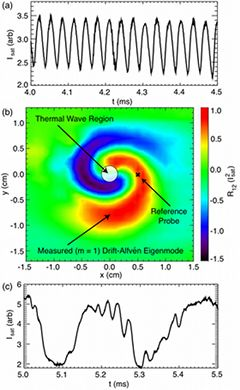Figure 2.14: Oscillations in ion saturation current due to (a) coherent drift-Alfvén mode and (c) thermal wave. (b) Two-dimensional contour of the cross-covariance, R12, between two probes with axial separation Δz = 160 cm.

Figure 2.14 provides a useful summary of the individual characteristics of the low frequency thermal waves and the higher-frequency drift-Alfvén waves. The time range sampled overlaps between the second and third stages identified in Fig. 2.13, before the broadband develops. The center panel is a two-dimensional (across the magnetic field) spatial domain that covers the full radial extent of the filament. The color contour represents the cross-covariance between$I_{sat}$ signals from Langmuir probes separated by an axial distance of Δz = 160 cm. One probe is fixed at a position z = 384 cm away from the beam injector and at a radial position within the region of measurable drift-Alfvén wave activity. The other probe is placed at z = 544 cm and it scans a two-dimensional plane across the confinement magnetic field. Measured signals are digitally band-pass filtered to isolate oscillations due to the drift-Alfvén modes. The cross-covariance is calculated at zero time delay for each plasma discharge over a restricted time interval that captures the largest drift-Alfvén wave. The resulting signal is averaged over 20 independent discharge pulses at every spatial location sampled. The result is an amplitude-dependent measurement of the phase difference between the signals of the two probes, and therefore contains an imprint of the spatial structure of the coherent drift-Alfvén wave at one time in the plasma discharge. The light circular region at the center of the drift-Alfvén wave depicts the highly localized thermal oscillation. The trace in the top panel of Fig. 2.14 shows the time evolution of the ion saturation current at a location r = 5 mm, i.e., within the gradient region. It shows the coherent oscillations associated with the two-dimensional snapshot seen in the center panel. These oscillations have typical frequencies in the range of 25-40 kHz. The bottom panel shows the temporal evolution of the ion saturation current at the center of the filament. It is clear that the oscillations here have much lower frequency (by a factor of 5-8) than those in the gradient region. A cross-modulation that shows the faint presence of the drift-Alfvén mode can be seen in portions of the trace.Figure 2.15: z = 384 cm (a) Radial temperature profile of the filament at t = 3.0 ms. (b) Two-dimensional contour of$I_{sat}$ illustrating the symmetric nature of the filament during early times.

Finally, at the latest times of the beam heating, 8 ≤ t ≤ 10 ms, the fluctuations become turbulent and there are no observable coherent modes in the spectra. Figure 2.13 illustrates that the amplitude of these fluctuations is large, exhibiting δTe/Te ≈ 50%. Transport is enhanced above classical levels, or “anomalous”, during this turbulent phase of the filament’s evolution.

##### 2.3.2 Spatial Behavior

Radial profiles at z = 384 cm are shown in Fig. 2.15. Panel (a) presents a profile at t = 3.0 ms during the classical transport stage. Within only 0.5 cm the filament has nearly reached a uniform temperature. Panel (b) is a two-dimensional contour of$I_{sat}$ that highlights the symmetric nature of the filament. This result is taken from t = 1.0 ms, also during the classical transport stage. Comparisons between the radial profiles during classical and anomalous transport regimes elucidate the difference between classical and anomalous thermal transport.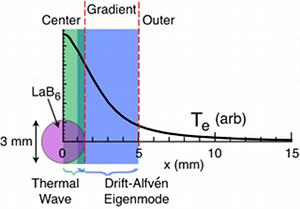Figure 2.16: Diagram highlighting the different spatial regimes in the radial profile of temperature. The thermal wave is confined to the center of the filament while the drift-Alfvén eigenmode is an extended structure. The drift-Alfvén mode exists as a global feature, but its amplitude peaks in the gradient region.

The radial symmetry shown in Fig. 2.15 is observed at all axial positions. This regularity during the early time evolution of the filament allows for the summary drawing given in Fig. 2.16. In this figure, a mapping of fluctuation behavior is given in terms of a typical radial temperature profile. The LaB6 crystal is shown for perspective. The thermal wave is strictly confined to the center of the filament, though it does overlap with the global structure of the drift-Alfvén eigenmode. In Fig. 2.16 the gradient region is labeled as the location of the drift-Alfvén wave because that is where it exhibits its largest amplitude.

##### 2.3.3 Spatiotemporal Evolution

Figure 2.17 illustrates the marked difference in the filament profile before and after the transition to anomalous transport. In panel (a) a cylindrically symmetric profile is observed at t = 1 ms. Well after the transition away from this classical regime, panel (b) presents the resulting profile at a time of t = 8 ms. Both of these contours are actually ensemble results over a few thousand discharges.Figure 2.17:$I_{sat}$ contours at z = 544 cm (a) The t = 1.0 ms time is the standard used to experimentally determine the filament center. (b) At t = 8 ms the filament has transitioned to a turbulent state in which both classical transport and cylindrical symmetry are no longer present.

#### 2.4 Relationships Between Physics Results and Filament Behavior

##### 2.4.1 Thermal Waves Appear in the Filament Center

The thermal waves discussed in Chapter 3 are only observed in the radial center of the filament. This is a result required by the nature of thermal waves because their drive source must be a heating source. While presently unknown, the heating source for the thermal waves must reside within the filament because the only energy source for all heating is the beam itself (the thermal wave may be excited by a heat source located axially away from the beam and does not have to be driven by the beam directly). Thermal waves are observed to initially appear at different times in the filament’s evolution.

##### 2.4.2 Exponential Spectra Occur in the Anomalous Transport Regime

The Lorentzian pulses and the resulting exponential spectra of Chapter 4 are observed at all spatial locations, but only late in time after the transition from classical to anomalous transport has occurred. This turbulent behavior is observed in many plasma devices, in all cases it appears driven by the pressure gradients existing in plasma boundaries (e.g., tokamak scrape-off layers and edge regions of linear devices).